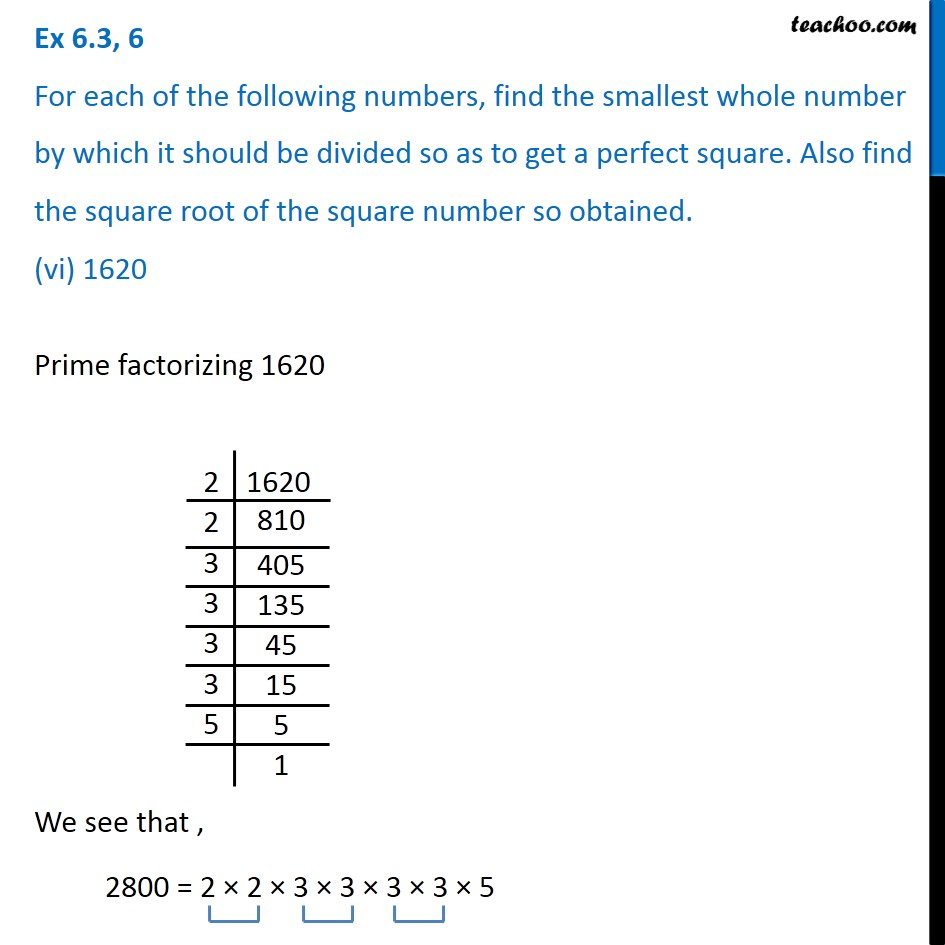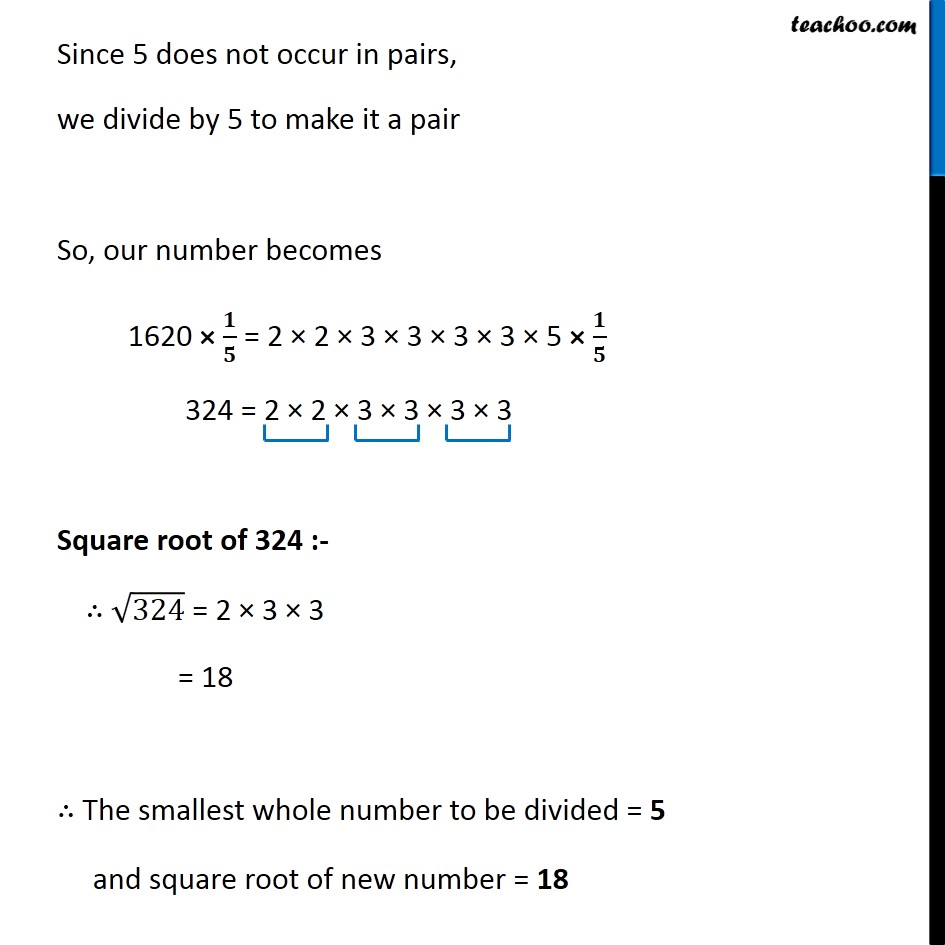Ex 6.3

Chapter 6 Class 8 Squares and Square Roots
Serial order wise### Transcript

Ex 6.3, 6 For each of the following numbers, find the smallest whole number by which it should be divided so as to get a perfect square. Also find the square root of the square number so obtained. (vi) 1620Prime factorizing 1620 We see that , 2800 = 2 × 2 × 3 × 3 × 3 × 3 × 5 Since 5 does not occur in pairs, we divide by 5 to make it a pair So, our number becomes 1620 × 𝟏/𝟓 = 2 × 2 × 3 × 3 × 3 × 3 × 5 × 𝟏/𝟓 324 = 2 × 2 × 3 × 3 × 3 × 3 Square root of 324 :- ∴ √324 = 2 × 3 × 3 = 18 ∴ The smallest whole number to be divided = 5 and square root of new number = 18